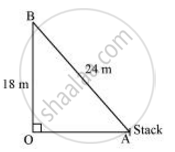Share

# A guy wire attached to a vertical pole of height 18 m is 24 m long and has a stake attached to the other end. How far from the base of the pole should the stake be driven so that the wire will be taut? - Mathematics

Course

#### Question

A guy wire attached to a vertical pole of height 18 m is 24 m long and has a stake attached to the other end. How far from the base of the pole should the stake be driven so that the wire will be taut?

#### SolutionLet OB be the pole and AB be the wire.

By Pythagoras theorem,

AB2 = OB2 + OA2

(24m)2 = (18m)2 + OA2

OA2 = (576 - 324)m2 = 25 m2

OA2 = sqrt252

Therefore, the distance from the base is 6sqrt7 m

Is there an error in this question or solution?

#### APPEARS IN

NCERT Solution for Mathematics Textbook for Class 10 (2019 (Latest))
Chapter 6: Triangles
Ex. 6.5 | Q: 10 | Page no. 151
RD Sharma Solution for Class 10 Maths (2018 (Latest))
Chapter 7: Triangles
Ex. 7.7 | Q: 20 | Page no. 121
RD Sharma Solution for Class 10 Maths (2018 (Latest))
Chapter 7: Triangles
Ex. 7.7 | Q: 20 | Page no. 121

#### Video TutorialsVIEW ALL 

Solution A guy wire attached to a vertical pole of height 18 m is 24 m long and has a stake attached to the other end. How far from the base of the pole should the stake be driven so that the wire will be taut? Concept: Pythagoras Theorem.
S